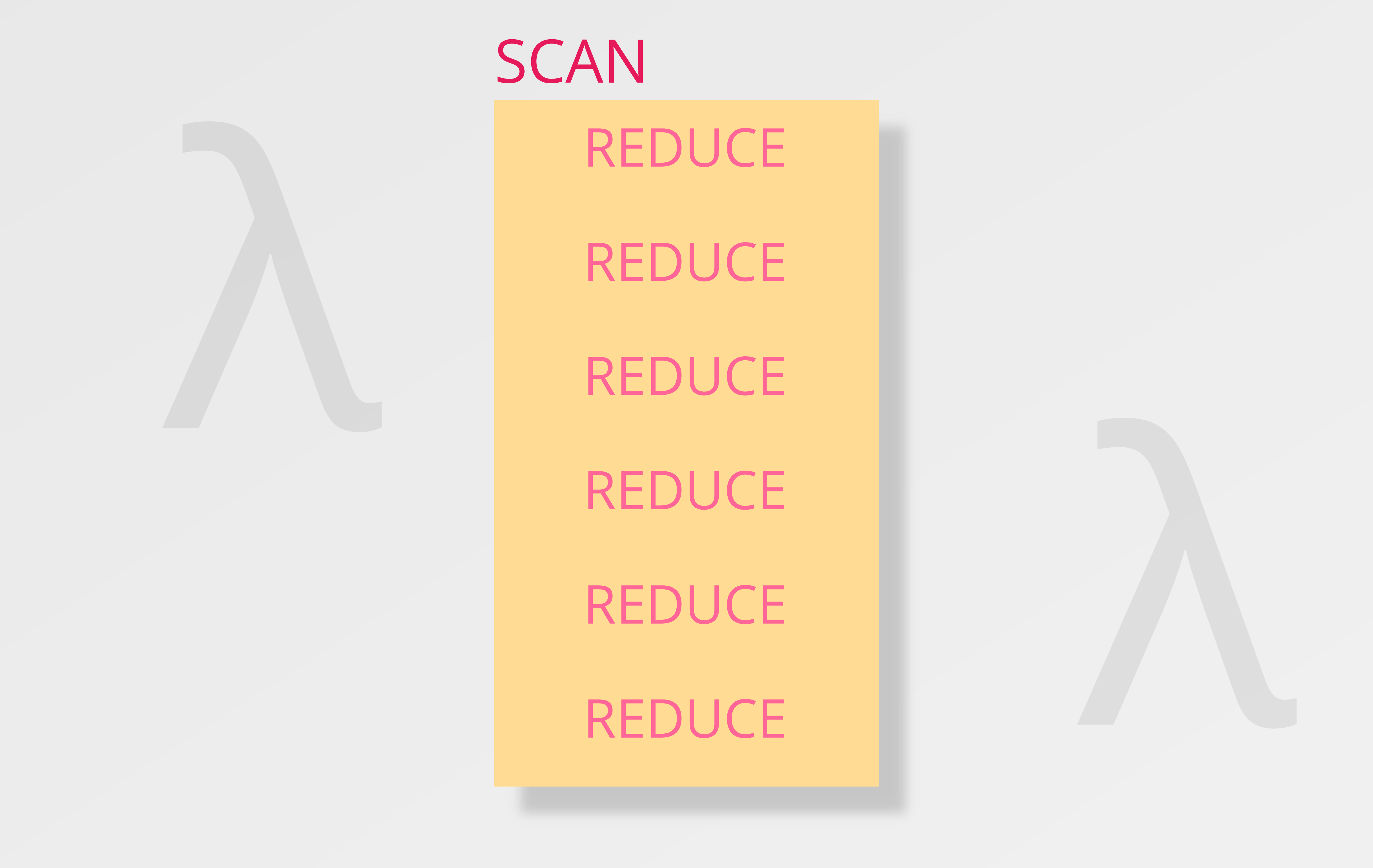## Scan function in just one line in TS

Praveenkumar
Aug 01, 2020### Prerequisite:

• Basic understanding of generic functions in typescript.
• Basic understanding of functional programming.

I will be using lodash library for some of the examples and to implement the one liner.

`scan` function is a higher order function similar to `reduce`, but `reduce` returns a single value whereas a `scan` returns an array of values. Lets see some examples to understand better.

REDUCE :

Passing `[1 , 2, 3, 4, 5]` to `reduce` function with identity element as `0` and `sum` as the input function will produce `15` as the output.

``````import { reduce } from 'lodash';

reduce([1, 2, 3, 4, 5], (a, b) => a + b, 0);

// Steps
0 + 1 = 1
1 + 2 = 3
3 + 3 = 6
6 + 4 = 10
10 + 5 = 15

// Output:
15
``````

`reduce` function takes the input function `(a, b) => a + b` and applies it on `0` (identity value) and `1`, then takes it output which is `1` and passes it as first parameter to the next function execution along with the next element in the array. This process is continued until the last element of the array and the result is returned.

SCAN :

Passing `[1 , 2, 3, 4, 5]` to `scan` function with identity element as `0` and `sum` as the input function will produce `[1, 3, 6, 10, 15]` as the output.

``````scan([1, 2, 3, 4, 5], (a, b) => a + b, 0);

// Steps
0 + 1 = 
1 + 2 = [1, 3]
3 + 3 = [1, 3, 6]
6 + 4 = [1, 3, 6, 10]
10 + 5 = [1, 3, 6, 10, 15]

// Output:
[1, 3, 6, 10, 15]
``````

As you can see from above code, similar to `reduce` function the `scan` function passes output of first function, as the input to the next function execution but the only difference being that, the result of each function execution is stored in an array and that array is returned as a result.

You can notice that, the last element of the resulting array is `15` which was the output of `reduce` function.

So the main idea here is,

For the same input array of values and identity element, the result of a reduce function is always the last element in the result of a scan function.

Lets implement the `scan` function now.

``````import { range, take, reduce } from "lodash";

const scan = <T>(values: T[], fn: (t1: T, t2: T) => T, identity: T): T[] =>
range(1, values.length + 1)
.map((i) => take(values, i))
.map((a) => reduce(a, fn, identity));
``````

Don't worry, if you don't understand the above code now. Lets breakdown the above code to understand.

• First parameter of the function is an `array` of type `T`.
• Second parameter of the function is a `function` that takes two parameters of type `T`.
• Last parameter of the function is an `identity` element of type `T`.
• The return type of the function is an `array` of elements of type `T`.

Now lets look at the body of the function. Body of the function is formatted differently here for better understanding.

``````range(1, values.length + 1)
.map((i) => take(values, i))
.map((a) => reduce(a, fn, identity));
``````

The range function creates a range iterator from `1` to `n`, where `n` is the length of the input array. From these numbers we use take function to take `i` elements from the `values` array each time. In other words, we make chunks of array from the given array, each holding `1`, `1..2`, `1..3` and so on until `1..n` values. This will create an array of arrays like below.

``````[, [1, 2], [1, 2, 3], [1, 2, 3, 4], [1, 2, 3, 4, 5]];
``````

When we apply `reduce` function on each of the element (array) of the array with the input `identity` as identity element, we will get the expected result like below.

``````[1, 3, 6, 10, 15];
``````

Hope you enjoyed! Happy Hacking!

functional-programmingjavascripttypescript

WRITTEN BY

### Praveenkumar

I am a passionate full stack developer. I develop primarily using JS specifically TS. I also work on JAVA and python. I like exploring latest languages, technologies and frameworks.